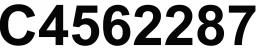# Equation: 3955

What is the value of x in this equation: 2.5x: 4 = 7.5. x is a natural number.

x =  12

### Step-by-step explanation:

2.5x/4=7.5

2.5•x/4=7.5

2.5x = 30

x = 12

Our simple equation calculator calculates it.Did you find an error or inaccuracy? Feel free to write us. Thank you!

Tips for related online calculators
Do you have a linear equation or system of equations and looking for its solution? Or do you have a quadratic equation?